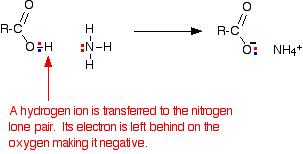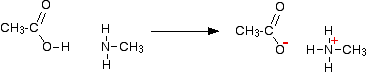Need help? Email help@shout.education

# Carboxylic Acids as Acids

This page looks at the simple reactions of carboxylic acids as acids, including their reactions with metals, metal hydroxides, carbonates and hydrogencarbonates, ammonia and amines.

Note: This page covers all the reactions likely to be asked by any of the UK syllabuses for 16 – 18 year olds, but no single syllabus is likely to want all of them. Check your syllabus and past papers to find out what you need to know. If you are a studying a UK-based syllabus and haven't got these, follow this link to find out how to get hold of them.

## The Acidity of the Carboxylic Acids

### Why Are Carboxylic Acids Acidic?

Using the definition of an acid as a "substance which donates protons (hydrogen ions) to other things", the carboxylic acids are acidic because of the hydrogen in the -COOH group.

In solution in water, a hydrogen ion is transferred from the -COOH group to a water molecule. For example, with ethanoic acid, you get an ethanoate ion formed together with a hydroxonium ion, H3O+.

This reaction is reversible and, in the case of ethanoic acid, no more than about 1% of the acid has reacted to form ions at any one time. (This is a rough-and-ready figure and varies with the concentration of the solution.)

These are therefore weak acids.

CH_3COOH + H_2O \xrightleftharpoons{} CH_3COO^- + H_3O^+

This equation is often simplified to:

CH_3COOH_{(aq)} \xrightleftharpoons{} CH_3COO^-_{(aq)} + H^+_{(aq)}

However, if you are going to use this second equation, you must include state symbols.They imply that the hydrogen ion is actually attached to a water molecule.

### The pH of Carboxylic Acid Solutions

The pH depends on both the concentration of the acid and how easily it loses hydrogen ions from the -COOH group.

Ethanoic acid is typical of the acids where the -COOH group is attached to a simple alkyl group. Typical lab solutions have pH's in the 2 – 3 range, depending on their concentrations.

Methanoic acid is rather stronger than the other simple acids, and solutions have pH's about 0.5 pH units less than ethanoic acid of the same concentration.

Note: You will find factors affecting the acidity of organic acids discussed in detail if you follow this link.

If you should know how to calculate the pH of weak acids like ethanoic acid, but aren't happy about it, you might be interested in my chemistry calculations book.

## Reactions of the Carboxylic Acids

### With Metals

Carboxylic acids react with the more reactive metals to produce a salt and hydrogen. The reactions are just the same as with acids like hydrochloric acid, except they tend to be rather slower.

For example, dilute ethanoic acid reacts with magnesium. The magnesium reacts to produce a colourless solution of magnesium ethanoate, and hydrogen is given off. If you use magnesium ribbon, the reaction is less vigorous than the same reaction with hydrochloric acid, but with magnesium powder, both are so fast that you probably wouldn't notice much difference.

2CH_3COOH_{(aq)} + \text{Mg}_{(s)} \longrightarrow (CH_3COO)_2Mg_{(aq)} + H_{2(g)}

Warning! Students have a high tendency to get the formulas of salts like magnesium ethanoate wrong. Remember that these simple carboxylic acids form ions with a single negative charge, and that the magnesium ion has two positive charges. In essence, this is no different from writing the formula for magnesium chloride – except that with these salts the metal is written at the end of the formula rather than at the beginning.

### With Metal Hydroxides

These are simple neutralisation reactions and are just the same as any other reaction in which hydrogen ions from an acid react with hydroxide ions. They are most quickly and easily represented by the equation:

H^+_{(aq)} + OH^-_{(aq)} \longrightarrow H_2O_{(l)}

Note: This assumes that the hydroxide is in solution. (It probably will be, because almost the only reaction ever done of this type is with sodium hydroxide solution!) If you were using a solid hydroxide, however, you would have to swap the hydroxide state symbol from "(aq)" to "(s)".

If you mix dilute ethanoic acid with sodium hydroxide solution, for example, you simply get a colourless solution containing sodium ethanoate. The only sign that a change has happened is that the temperature of the mixture will have increased.

This change could well be represented by the ionic equation above, but if you want it, the full equation for this particular reaction is:

CH_3COOH_{(aq)} + \text{Na}OH_{(aq)} \longrightarrow CH_3COO\text{Na}_{(aq)} + H_2O_{(l)}

### With Carbonates and Hydrogencarbonates

In both of these cases, a salt is formed together with carbon dioxide and water. Both are most easily represented by ionic equations.

##### For carbonates:
2H^+_{(aq)} + {CO_3}^{2-}_{(s)} \longrightarrow H_2O_{(l)} + CO_{2(g)}
##### and for hydrogencarbonates:
H^+_{(aq)} + {HCO_3}^-_{(s)} \longrightarrow H_2O_{(l)} + CO_{2(g)}

Note: This time, this assumes that the carbonate or hydrogencarbonate will be a solid – which it often is. If you used a solution of, say, sodium carbonate or hydrogencarbonate, you would have to remember to change the state symbol.

If you pour some dilute ethanoic acid onto some white sodium carbonate or sodium hydrogencarbonate crystals, there is an immediate fizzing as carbon dioxide is produced. You end up with a colourless solution of sodium ethanoate.

##### With sodium carbonate, the full equation is:
2CH_3COOH_{(aq)} + \text{Na}_2CO_{3(s)} \longrightarrow 2CH_3COO\text{Na}_{(aq)} + H_2O_{(l)} + CO_{2(g)}
##### and for sodium hydrogencarbonate:
CH_3COOH_{(aq)} + \text{Na}HCO_{3(s)} \longrightarrow CH_3COO\text{Na}_{(aq)} + H_2O_{(l)} + CO_{2(g)}

There is very little obvious difference in the vigour of these reactions compared with the same reactions with dilute hydrochloric acid.

However, you would notice the difference if you used a slower reaction – for example with calcium carbonate in the form of a marble chip. With ethanoic acid, you would eventually produce a colourless solution of calcium ethanoate.

2CH_3COOH_{(aq)} + \text{Ca}CO_{3(s)} \longrightarrow (CH_3COO)_2\text{Ca}_{(aq)} + H_2O_{(l)} + CO_{2(g)}

In this case, the marble chip would react noticeably more slowly with ethanoic acid than with hydrochloric acid.

### With Ammonia

Ethanoic acid reacts with ammonia in exactly the same way as any other acid does. It transfers a hydrogen ion to the lone pair on the nitrogen of the ammonia and forms an ammonium ion.If you mix together a solution of ethanoic acid and a solution of ammonia, you will get a colourless solution of ammonium ethanoate.

CH_3COOH_{(aq)} + NH_{3(aq)} \longrightarrow CH_3COONH_{4(aq)}

### With Amines

Amines are compounds in which one or more of the hydrogen atoms in an ammonia molecule have been replaced by a hydrocarbon group such as an alkyl group. For simplicity, we'll just look at compounds where only one of the hydrogen atoms has been replaced. These are called primary amines.

##### Simple primary amines include:

The small amines are very similar indeed to ammonia in many ways. For example, they smell very much like ammonia and are just as soluble in water. Because all you have done to an ammonia molecule is swap a hydrogen for an alkyl group, the lone pair is still there on the nitrogen atom.

That means that they will react with acids (including carboxylic acids) in just the same way as ammonia does.

For example, ethanoic acid reacts with methylamine to produce a colourless solution of the salt methylammonium ethanoate.Note: I have deliberately not tried to write this as a one line equation. It is much easier to see what is happening, to write it, and to remember it, if you draw the structures.

Don't be put off by the name of the product – methylammonium ethanoate. "Methylammonium" just means an ammonium ion in which one of the hydrogens is replaced by a methyl group.

However complicated the amine, because all of them have got a lone pair on the nitrogen atom, you would get the same sort of reaction.

Note: If you want to find out more about amines you could explore the amines menu by following this link.